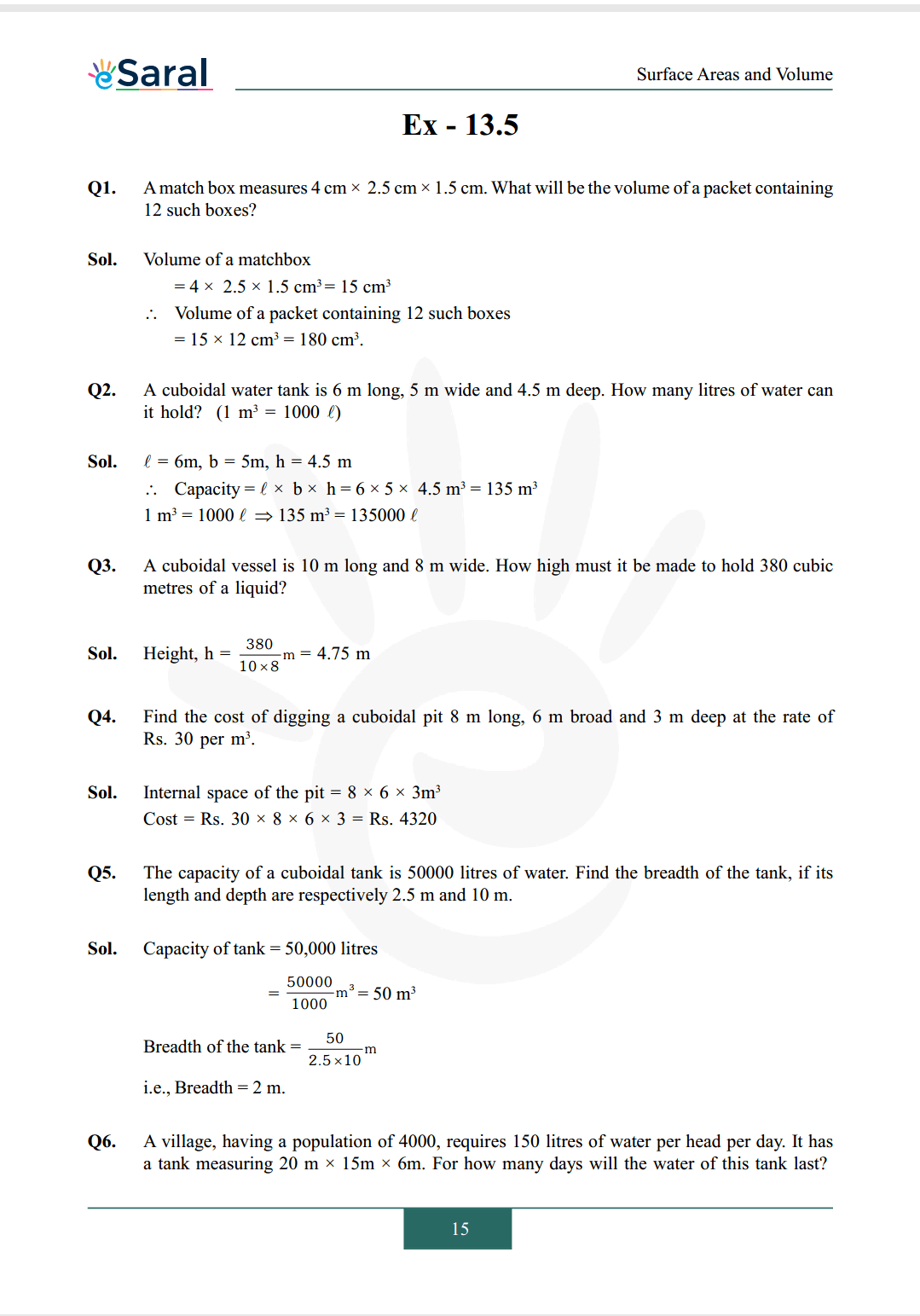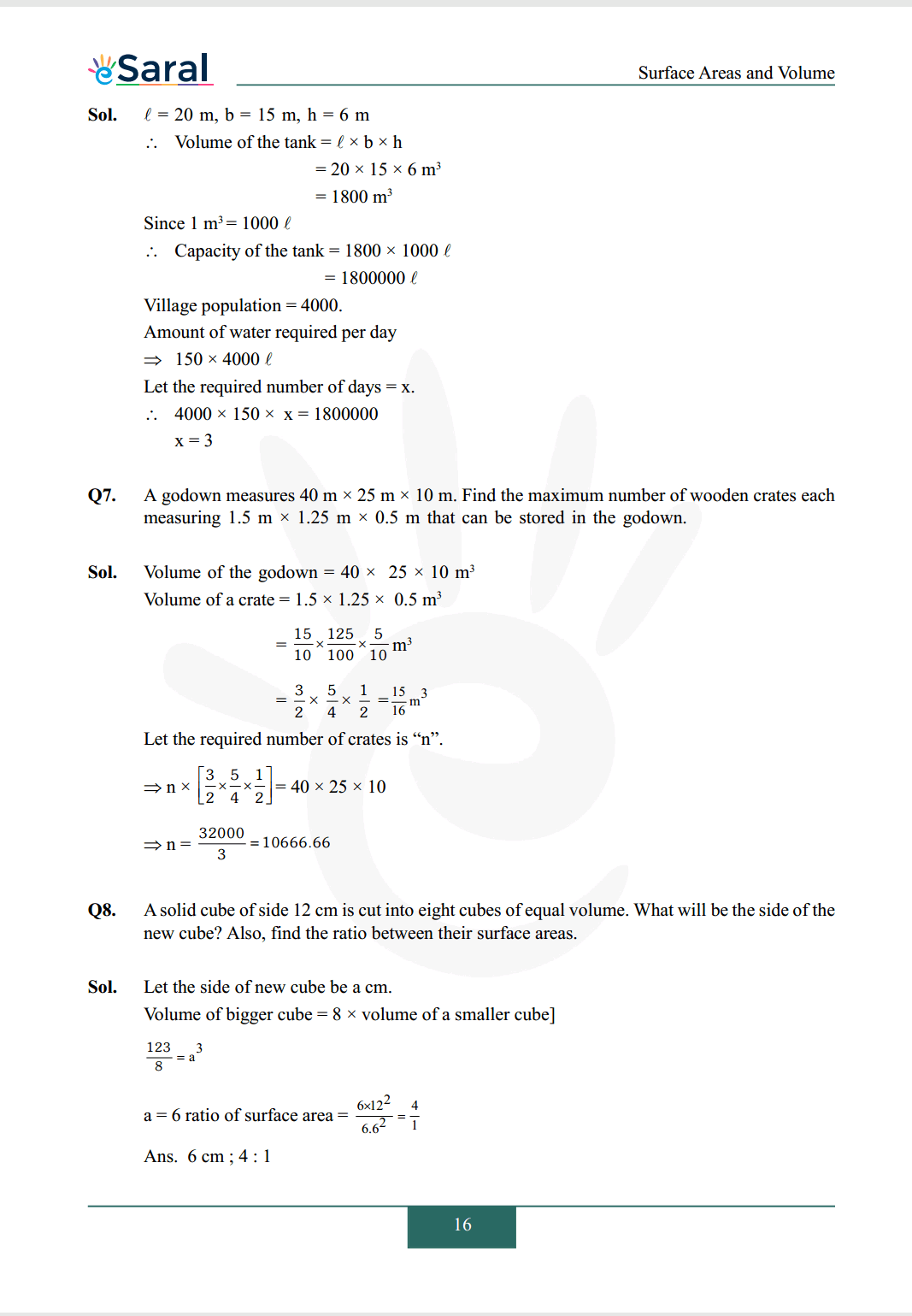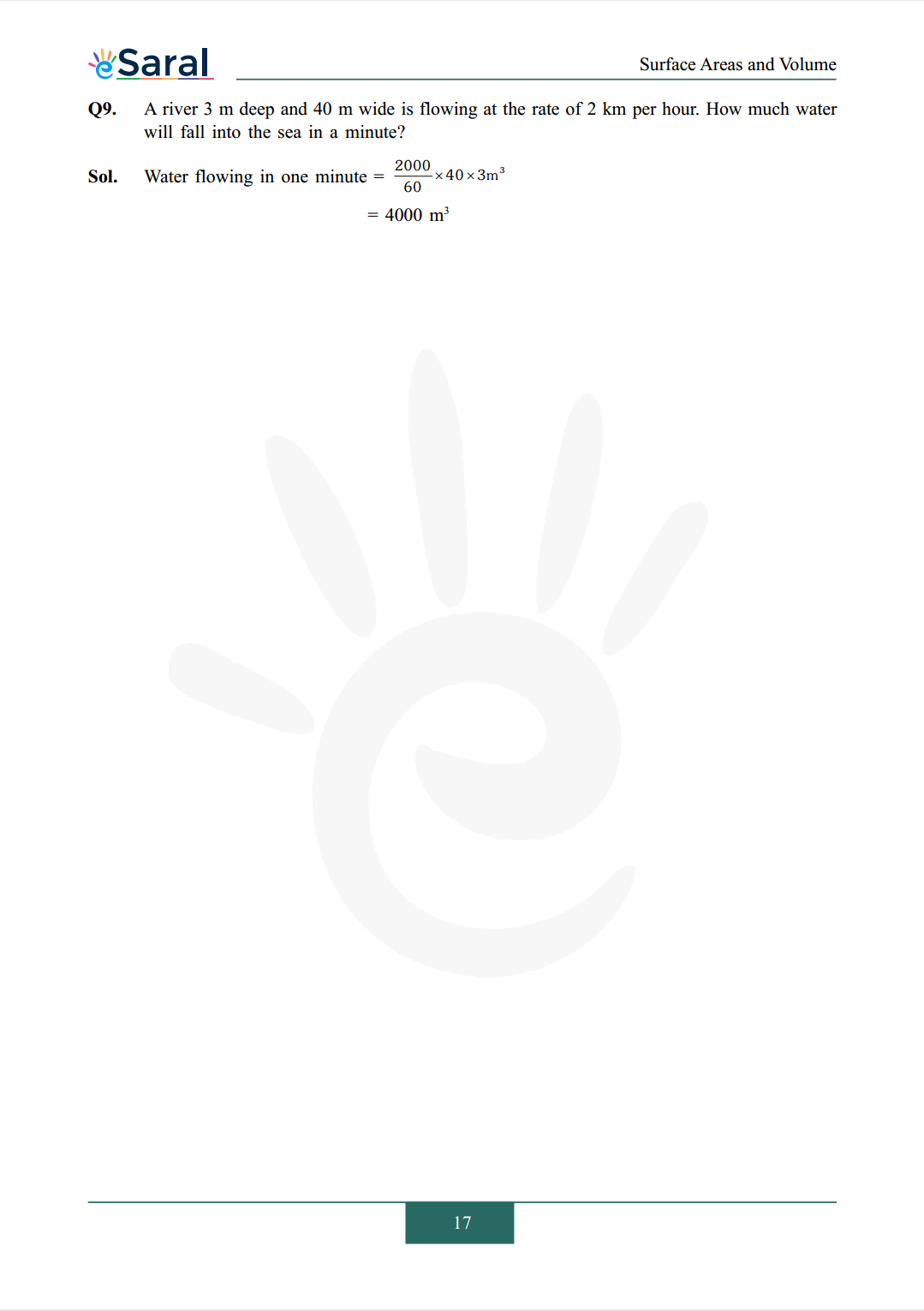Most Affordable JEE | NEET | 8,9,10 Preparation by Kota's Top IITian Doctor Faculties

# NCERT Solutions for Class 9 Maths chapter 13 Exercise 13.5 - Surface Areas and VolumesHey, are you a class 9 Student and Looking for Ways to Download NCERT Solutions for Class 9 Maths chapter 13 Exercise 13.5? If Yes then you are at the right place.

Here we have listed Class 9 maths chapter 13 exercise 13.5 solutions in PDF that is prepared by Kota’s top IITian’s Faculties by keeping Simplicity in mind.

If you want to score high in your class 9 Maths Exam then it is very important for you to have a good knowledge of all the important topics, so to learn and practice those topics you can use eSaral NCERT Solutions.

So, without wasting more time Let’s start.

### Download The PDF of NCERT Solutions for Class 9 Maths chapter 13 Exercise 13.5 "Surface Areas and Volumes"#### All Questions of Chapter 13 Exercise 13.5

Once you complete the chapter 13 then you can revise Ex. 13.5 by solving following questions

Q1. Amatch box measures $4 \mathrm{~cm} \times 2.5 \mathrm{~cm} \times 1.5 \mathrm{~cm}$. What will be the volume of a packet containing 12 such boxes?

Q2. A cuboidal water tank is $6 \mathrm{~m}$ long, $5 \mathrm{~m}$ wide and $4.5 \mathrm{~m}$ deep. How many litres of water can it hold? $\quad\left(1 \mathrm{~m}^{3}=1000 \ell\right.$ )

Q3. A cuboidal vessel is 10 m long and 8 m wide. How high must it be made to hold 380 cubic meters of a liquid?

Q4. Find the cost of digging a cuboidal pit $8 \mathrm{~m}$ long, $6 \mathrm{~m}$ broad and $3 \mathrm{~m}$ deep at the rate of Rs. 30 per $\mathrm{m}^{3}$.

Q5. The capacity of a cuboidal tank is 50000 litres of water. Find the breadth of the tank, if its length and depth are respectively $2.5 \mathrm{~m}$ and $10 \mathrm{~m}$.

Q6. A village, having a population of 4000 , requires 150 litres of water per head per day. It has a tank measuring $20 \mathrm{~m} \times 15 \mathrm{~m} \times 6 \mathrm{~m}$. For how many days will the water of this tank last?

Q7. A godown measures $40 \mathrm{~m} \times 25 \mathrm{~m} \times 10 \mathrm{~m}$. Find the maximum number of wooden crates each measuring $1.5 \mathrm{~m} \times 1.25 \mathrm{~m} \times 0.5 \mathrm{~m}$ that can be stored in the godown.

Q8. A solid cube of side 12 cm is cut into eight cubes of equal volume. What will be the side of the new cube? Also, find the ratio between their surface areas.

Q9. A river $3 \mathrm{~m}$ deep and $40 \mathrm{~m}$ wide is flowing at the rate of $2 \mathrm{~km}$ per hour. How much water will fall into the sea in a minute?